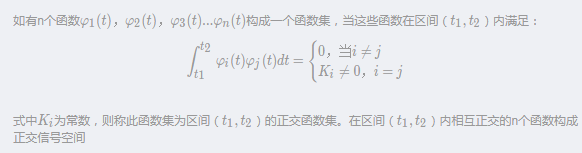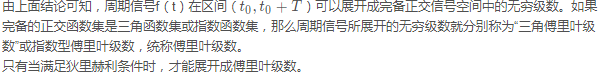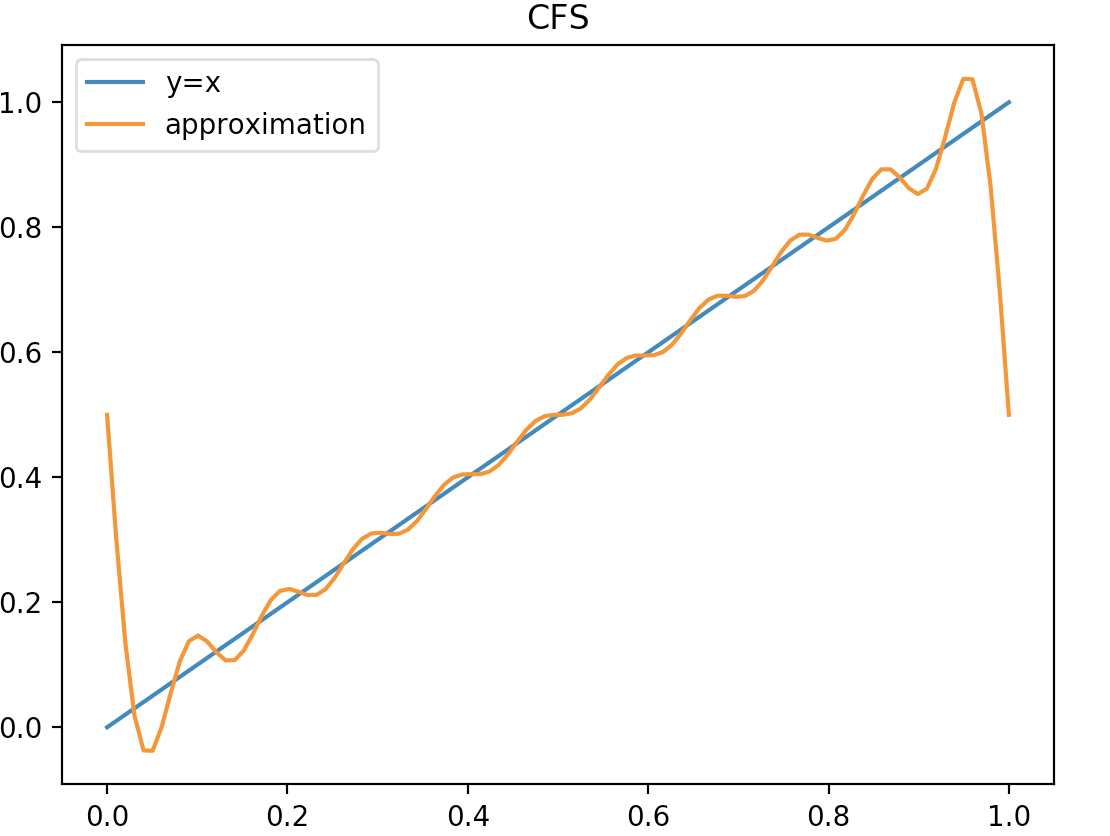• 傅里叶系数的推导
2020-12-23 23:48:17

不客气地说，

这个公式可以说是像“臭婆娘的裹脚布——又臭又长”，

而且来历相当蹊跷，

不知那个傅里叶什么时候灵光乍现，把一个周期函数

f(t)

硬生生地写成这么一大堆东西。单看

那个①式，

就是把周期函数

f(t)

描述成一个常数系数

a0

1

ω

sin

cos

函数、

2

ω

sin

cos

函数等、

n

ω

sin

cos

函数等一系列式子的和，

且每项都有不同的系数，

An

Bn

，至于这些系数，需要用积分来解得，即②③④式，不过为了积分方便，积分区间一

般设为

[-

π

,

π

]

，也相当一个周期

T

的宽度。

能否从数学的角度推导出此公式，

以使傅里叶级数来得明白些，

让我等能了解它的前世今生

呢？下面来详细解释一下此公式的得出过程：

１、把一个周期函数表示成三角级数：

首先，

周期函数是客观世界中周期运动的数学表述，

如物体挂在弹簧上作简谐振动、

单摆振

动、无线电电子振荡器的电子振荡等，大多可以表述为：

f(x)=A sin(

ω

t+

ψ

)

这里

t

表示时间，

A

表示振幅，

ω

为角频率，

ψ

为初相(与考察时设置原点位置有关)。

更多相关内容
• ## 傅里叶系数

千次阅读 2019-04-02 21:56:54
正交：向量点积后结果为0则说明两向量正交，比如 a(a1,a2,a3)a(a_1,a_2,a_3)a(a1​,a2​,a3​)与 b(b1,b2,b3)b (b _1,b_2,b_3)b(b1​,b2​,b3​)正交， 即a1b1+a2b2+a3b3=0a_1b_1+a_2b_2+a_3b_3=0a1​b1​+a2​b2​+...

以下均为不严格的，带个人理解的语言描述

1.三角函数系正交
正交：向量点积后结果为0则说明两向量正交，比如 a ( a 1 , a 2 , a 3 ) a(a_1,a_2,a_3) b ( b 1 , b 2 , b 3 ) b (b _1,b_2,b_3) 正交，
a 1 b 1 + a 2 b 2 + a 3 b 3 = 0 a_1b_1+a_2b_2+a_3b_3=0 ==>向量点积是对应分量相乘再累加
书本P116正交定义==>三角函数系的正交定义：比如cosx与sinx正交写为 ∫ T c o s x s i n x d x = 0 \int_{T} cosxsinx dx=0
可以理解为cosx，sinx在不同点上相乘再累加（积分）
定理：三角函数中任意三角函数（除本身）正交

2.既然三角函数彼此正交，那么可以将三角函数看成一个向量空间
书本P116正交函数集定义则f（x）周期（2 π \pi ）（满足狄利克雷条件下）可以拆分成
f （ x ） = a 0 + a 1 c o s x + b 1 s i n x + a 2 c o s 2 x + b 2 s i n 2 x + . . . a n c o s n x + b n s i n n x f（x）=a_0+a_1cosx+b_1sinx+a_2cos2x+b_2sin2x+...a_ncosnx +b_nsinnx
(理解为 a ⃗ = a i ⃗ + b j ⃗ + c k ⃗ \vec a=a\vec i+b\vec j+c\vec k )
物理意义：一个周期函数可以拆分成周期自身整数倍的三角函数线性组合。

书上P120注：狄利克雷条件：（1 ）在一周期内，连续或只有有限个第一类间断点；（2）在一周期内，极大值和极小值的数目应是有限个；（3）在一周期内，信号是绝对可积的。

3.系数推导
方法：消项
(1).求 a 0 a_0
如求 a 0 a_0 ,即将 a 0 a_0 之外的全部消掉
a 0 a_0 是不包含三角函数的系数，而其他都包含，周期都可为2 π \pi
所以只需对整体积分
∫ − π π f ( x ) d x = ∫ − π π ( a 0 + a 1 c o s x + b 1 s i n x + a 2 c o s 2 x + b 2 s i n 2 x + . . . a n c o s n x ) d x \int_{-\pi}^{\pi} f(x) dx=\int_{-\pi}^{\pi}(a_0+a_1cosx+b_1sinx+a_2cos2x+b_2sin2x+...a_ncosnx) dx
∫ − π π f ( x ) d x = ∫ − π π a 0 d x \int_{-\pi}^{\pi} f(x) dx=\int_{-\pi}^{\pi}a_0dx
a 0 = 1 2 π ∫ − π π f ( x ) d x a_0=\frac{1}{2\pi}\int_{-\pi}^{\pi}f(x)dx
(有的地方写 a 0 2 \frac{a_0}{2} 是一样的)

(2).
同样方法求an
方法：消项，三角函数正交
如求 a n c o s n x a_ncosnx ,将f(x)整体乘cosnx，再积分
∫ − π π c o s n x f ( x ) d x = ∫ − π π ( a 0 + a 1 c o s x + b 1 s i n x + . . . a n c o s n x ) c o s n x d x \int_{-\pi}^{\pi} cosnxf(x) dx=\int_{-\pi}^{\pi}(a_0+a_1cosx+b_1sinx+...a_ncosnx)cosnx dx
∫ − π π c o s n x f ( x ) d x = ∫ − π π a n c o s 2 n x d x \int_{-\pi}^{\pi} cosnxf(x) dx=\int_{-\pi}^{\pi}a_n cos^2nxdx
∫ − π π c o s n x f ( x ) d x = a n ∫ − π π c o s 2 n x + 1 2 d x \int_{-\pi}^{\pi} cosnxf(x) dx=a_n\int_{-\pi}^{\pi} \frac{cos2nx+1}{2}dx
∫ − π π c o s n x f ( x ) d x = a n π \int_{-\pi}^{\pi} cosnxf(x) dx=a_n\pi
a n = 1 π ∫ − π π c o s n x f ( x ) d x a_n=\frac{1}{\pi}\int_{-\pi}^{\pi} cosnxf(x) dx (推毕)

(3)
b n b_n 推导方法相同

展开全文• 用直接计算傅里叶系数的方法算法及练习，周期信号的频谱分析
• 傅里叶级数是周期信号的时域表达式，而傅里叶变换是非周期信号或周期信号的频谱(频域函数)，要想了解它们之间的关系，需要你耐心看完下面...(5)傅里叶级数、傅里叶系数傅里叶变换的关系是什么？(6)周期信号傅里...

傅里叶级数是周期信号的时域表达式，而傅里叶变换是非周期信号或周期信号的频谱(频域函数)，要想了解它们之间的关系，需要你耐心看完下面内容。

学过"信号与系统"等课程的人往往会被许多问题所困惑，如：

(1)周期信号傅里叶级数表示什么内容？

(2)信号的频谱表示什么？

(3)通过信号的频谱我们能知道什么？

(4)信号的时域和频域的关系是什么？

(5)傅里叶级数、傅里叶系数、傅里叶变换的关系是什么？

(6)周期信号傅里叶级数中的傅里叶系数物理意义是什么？

(7)周期信号傅里叶级数中的傅里叶系数与非周期信号傅里叶变换的关系是什么？

(8)非周期信号的傅里叶变换到底是什么意思？

(9)傅里叶变换的物理意义是什么？

(10)复数形式的傅里叶变换的物理意义？

(11)为什么周期信号的傅里叶变换在相应频率处出现冲激函数？

(12)为什么正弦(或余弦)信号的傅里叶变换是冲激函数？

上述问题尽管看上去有些零碎，其实它们是有联系的，下面，我从头到尾把这些问题串起来，内容可能比较多，如果你想知道结果，则需要你耐心阅读，并希望下面的内容能对你有所帮助，更详细的内容和应用还请参见我写的《信号与系统分析和应用》一书，本书在高等教育出版社出版发行。

如果你手里有《信号与系统分析和应用》教材，请你关注“信号与系统分析”微信公众号，那里面列出书中发现的问题。

要知道傅里叶变换把时域信号变换为频域函数(频谱)，首先需要知道信号的频谱是什么。我在教学的时候，规定时域是“信号”，频域是“函数”。

注意，下面我站在求解“频谱”的角度来说问题！

一、周期信号及其频谱

1、先从周期信号说起

周期信号的频谱表示了这个周期信号含有的所有不同频率余弦信号的频率、幅度和初相位这三个“参数”，每个余弦的这“三个参数”表征了这个余弦的全部信息，信号的频谱是用原周期信号含有的所有各个频率余弦信号的“三参数”来表征原时域信号的组成成分和分量(傅里叶级数是在时域用余弦信号的形式来表征周期信号的组成。注意：傅里叶级数是时域的，它的自变量是时间t)。

我们不能总是喋喋不休地只讨论一个复杂的时间信号是由哪些基本信号合成的，而我们真正要关心的是这个复杂信号的“组成成分”和这些“成分的分量”。我非常赞赏网友用的“配方”这个词，它一针见血地指出了一个混合物(相当于时域信号)和它的组成成分及其分量(频谱---信号配方)。可以看到，周期信号的“配方”就是组成这个周期信号的各个频率的余弦的“频率”、“幅度”和“初相位”这“三个参数”。

如一副中药相当于原时域信号，而它的“药单”相当于其“频谱”。

一副混合好的中药(相当于一个复杂信号)，你从下面图中看不出组成它的各成分的分量。一副混合好的中药(相当于一个复杂信号)，你看不出组成它的各成分的分量

要想知道它的组成成分和分量，你一定要拿到它的药单。药单上列出了一副中药的组成成分和各味药的“分量”。对应我们讨论的信号来说，药名相当于“余弦信号的频率”，重量相当于“余弦信号的幅度”。可以看到，这个药单图只有“各个味药”和它的重量，这个药单其实相当于“信号的幅度谱”。我们也可以把各味药的产地标上(也可以理解为余弦信号的初相位)。反过来，我们按着“药单”去抓药就能构成一副中药。

2、傅里叶积分公式(对非周期信号来说就是它的傅里叶变换)的伟大之处在哪里？

在这里为什么我要说“傅里叶积分公式”而不说“傅里叶变换”？因为，求周期信号的频谱是用傅里叶积分公式，而求非周期信号的频谱的公式我们通常称其为“傅里叶变换”，其实，傅里叶变换也是傅里叶积分公式。

傅里叶积分公式的伟大之处在于：利用信号的“正交性”，通过积分公式能求出原信号的“配方”或者说求出组成原信号所有不同频率余弦(或正弦)信号的“三参数”，也就是我们在信号与系统课程中讲到“频谱”。

傅里叶积分公式要完成两个任务：第一个是利用信号的“正交性”，从一个“混合物”(一个复杂信号)中分离出其中的一个成分(某个频率的余弦)，另一个是它像一杆秤似的称出被分离出来的那个成分的“分量”(余弦的幅度和初相位)。我们不但要知道一个混合物的“成分”，还要知道其中某个成分的“分量”。所以，傅里叶积分公式兼有“成分分离器”和“秤”的双重作用。

下面就让我们去看看如何从复杂信号中分离出一个余弦，然后怎样求出被分离出来的这个余弦的幅度和初相位(这是一个真正伟大的工作)。

3、周期信号的表示以及它的频谱的求解

我们先看看一个周期信号的时域表示(傅里叶级数)，然后就让我们去见证一个伟大的傅里叶积分公式，它是如何求出这个周期信号的“配方”(频谱)，也就是用傅里叶积分公式如何从周期信号中分离出一个余弦以及怎样求出这个余弦的幅度和初相位的(这是一个真正伟大的工作)。

(1)周期信号三角函数形式的傅里叶级数

为了尽快完成下面内容，下面我把我写的《信号与系统分析和应用》书上内容直接复制过来，更详细内容还请参见这本书。

请注意：为什么我把周期信号三角函数形式的傅里叶级数写成下面的形式，而不是公式(4.2-8)的形式？因为只有这样才能充分理解信号频谱以及频谱的作用、傅里叶系数、非周期确知信号的傅里叶变换的物理意义，才能充分理解我写的下面的内容。特别要注意：所谓的傅里叶级数是在时域用不同频率的余弦信号或正弦信号来表示原周期信号的组成。

由公式(4.2.2)可知：这样就能计算出一个周期信号的频谱了(“配方”或“药单”)。我们将所有“三参数”按频率的位置表示出来就是原周期信号的“频谱”了，因此，下面的周期信号的傅里叶级数公式才是与“频谱”对应的周期信号三角函数形式的傅里叶级数。用上面公式表示周期信号三角函数形式的傅里叶级数才能更好地理解信号的“频谱”到底表示了什么？以及后面我要说的非周期信号傅里叶变换的“物理意义”是什么，才能更好理解信号频域分析的目的。那么，公式(4.2-8)可以看做求解信号频谱的中间环节，当然，它也是三角函数形式的傅里叶级数，只是用它不利于理解信号频谱表示的内容(也有特殊情况)。

可以说，公式(4.2-10)以及(4.2-11)是“最伟大的积分公式”之一。这两个公式为什么能计算出an和bn？我们需要讨论信号的正交性问题。

下面把《信号与系统分析和应用》书上内容复制过来。注意：上面积分区间一定在是整倍周期期间才成立。这样，下面的积分公式的物理意义就很清楚了：想必大家已经领略到了数学的伟大魔力了吧。

4、周期信号组成成分的表示---信号频谱可以看到，频谱图(a)和(b)表示了组成原周期信号的所有不同频率余弦信号的“频率”(横坐标)、“幅度”以及“初相位”这三个参数，这与公式(4.2.2)是对应的，这就是为什么我将周期信号傅里叶级数写成公式(4.2.2)的根本原因。

信号频谱的作用就是用图形(频谱图)或公式(向量形式)来表示组成这个周期信号的所有不同频率的余弦信号的“三参数” (幅度、初相和频率或角频率)，也就是说，频谱是用“参数”的形式表示原信号的组成成分，我们不但要知道信号的组成成分还要知道这些成分的份额，这就是大家说到的“原信号的配方”。从频谱图上，我们就能看到原周期信号含有的所有频率的余弦(或正弦)信号的幅度和相位的大小，也就知道了周期信号含有的所有频率成分以及这些频率成分对原信号的贡献大小。上面图(c)是将图(a)和(b)合成一个图(合成的原则请参见《信号与系统分析和应用》书)。

周期信号的傅里叶级数是在时域用不同频率的余弦信号或正弦信号来表示原周期信号的组成，而周期信号的频谱是用“参数”的形式表示这个周期信号的组成成分。

5、周期信号复指数形式的傅里叶级数与信号频谱周期信号复指数形式傅里叶级数中的傅里叶系数Xn是用复数的形式表示每个余弦信号的幅度和初相位信息(包含余弦信号的两个参数)。

一定注意：傅里叶系数Xn的积分公式其实还是求an和bn(系数为0.5)，只是用一个积分公式的实部和虚部一起求出的，它还是利用“正交性”，傅里叶系数Xn就是复数形式的原周期信号的“频谱”(“药单”或“配方”)。

特别强调一下：将傅里叶系数Xn的虚部看成是余弦信号的初相是不对的，它的虚部0.5bn是“正弦分量”的幅度信息，不是余弦信号的初相位，而余弦信号的初相是公式(4.4-17)，下面讨论非周期信号傅里叶变换也是这个问题，即绝对可积非周期信号的傅里叶变换是一个复函数，它的虚部也不是任意角频率w的余弦信号的初相位。

二、非周期信号的傅里叶变换

非周期信号的傅里叶变换是从周期信号复指数形式傅里叶级数中的傅里叶系数Xn推导来的(注意：不是从傅里叶级数推导来的！)，所以，非周期信号的傅里叶变换就是非周期信号的“频谱”。

绝对可积信号的傅里叶变换是自变量为频率或角频率的复函数，它含有原时域信号含有的所有频率余弦信号的“三参数”信息(频率信息是由傅里叶变换的自变量来表征的)，它是一个复函数(他跟周期信号傅里叶级数中的傅里叶系数Xn的物理意义相似)，它的实部表示原信号含有的任意角频率w余弦信号的同相分量(余弦分量)的幅度信息，其虚部表示信号含有的任意角频率w余弦信号的正交分量(正弦分量)的幅度信息。但是，绝对可积非周期信号含有的每个余弦信号的幅度都趋于无穷小，非周期信号的傅里叶变换中的幅度谱是每个余弦信号无穷小的幅度乘上一个无穷大的周期。如果一个非周期信号是确知信号，则它的傅里叶变换就是一个自变量为频率或角频率的确知相量函数(所以，不能把它叫做信号)，这说明，这个原确知时间信号含有的所有频率余弦信号的幅度和初相不是孤立的，他们满足一定关系，这个关系就是以自变量为频率或角频率的“频域函数”。更多内容请参看我写的《信号与系统分析和应用》书上第4章和第5章内容。下面举个信号的例子：上面是信号傅里叶变换是复函数的物理意义。下面看看因果稳定系统的频率响应的物理意义。

因果稳定系统的频率响应是此系统单位冲激响应的傅里叶变换，由于此系统是因果稳定系统，则其频率响应也是复函数。可以看到，信号的傅里叶变换与系统单位冲激响应的傅里叶变换即使都是复函数，但是，它们的物理意义是不同的。

三、周期信号的傅里叶变换以及冲激函数的作用除了上述对信号进行傅里叶变换得到信号的频谱以及对系统单位冲激响应进行傅里叶变换而得到系统频率响应，这些“傅里叶变换”都有其物理意义，人们还发现时域信号经过傅里叶变换后在变换域内其频域函数之间的运算比时域简单，人们借助于频域运算可以简化时域里的运算。最后，简单总结一下傅里叶变换：

(1)对信号进行傅里叶变换得到信号的频谱；

(2)对系统单位冲激响应进行傅里叶变换得到系统频率响应；

(3)经过傅里叶变换后能使运算简单；

如果你手里有《信号与系统分析和应用》教材，请你关注“信号与系统分析”微信公众号，那里面列出书中发现的问题。

展开全文• 计算机网络课程讲到物理层，布置作业的第一题是求f(t)=t (0≤t≤1)的傅里叶系数。 　我们知道任何一个周期函数都可以被傅里叶级数逼近。如果是实值函数，则可以用正弦分量，余弦分量，直流分量来近似。公式如下：$$... 计算机网络课程讲到物理层，布置作业的第一题是求f(t)=t (0≤t≤1)的傅里叶系数。 我们知道任何一个周期函数都可以被傅里叶级数逼近。如果是实值函数，则可以用正弦分量，余弦分量，直流分量来近似。公式如下：$$f(x)=\frac{a_0}{2}+\sum_{n=1}^{\infty}(a_n cosnx+b_n sinnx)

利用正余弦函数的正交性反变换可得到f(x)的系数。本题中认为函数周期是1，进行延拓可以看成是无数个f(t)沿着x轴平移。

简单计算得$a_0=1$, $a_n=0$, $b_n=-\frac{1}{\pi n}$ 至此题目结束。

但是我想练习一下最近自己刚刚接触到的numpy和matplotlib，于是动手写了一个python小程序想看看拟合的效果是什么样的。效果图和代码如下。

import numpy as np
import matplotlib.pyplot as plt

x = np.linspace(0, 1, 100)
y_ = np.zeros_like(x)
y = x
y_ = y_ + 0.5
for n in range(1, 11):
y_ = y_ - 1/np.pi/n * np.sin(2*np.pi*x*n)

plt.plot(x, y, label='y=x')
plt.plot(x, y_, label='approximation')

plt.xlabel('x')
plt.ylabel('y')

plt.title("CFS")
plt.legend()
plt.show()代码一直拟合到十阶分量。可看到效果还是不错的，增加x采样点可以增加图像平滑

展开全文• ——————评论区小伙伴的补充——————感谢评论区的朋友 @摩天轮1111 指出合成公式的满足条件，在特定情况下合成公式不一定成立。...原答案解释了傅里叶级数分析公式(傅里叶系数求解公式)的由来...
• Y = Fseriesval(a,b,X) 计算由系数 a 和 b 在向量 X 中的值定义的傅立叶级数。 额外的参数允许重新缩放 X 数据和仅正弦或仅余弦的扩展。 例子： % 生成数据x = linspace(0,2,41)'; y = mod(2*x,1); % 使用 F...matlab
• 如果一个函数的傅里叶级数处处收敛于 f(x)f(x)f(x)，则称这个级数是这个函数的傅里叶展开式，即： f(x)=a02+∑n=1∞an(cos⁡nx+bnsin⁡nx),x∈[−π,π] f(x)=\frac{a_{0}}{2}+\sum_{n=1}^{\infin}a_{n}(\cos{nx}+b_...
• 傅里叶级数系数 的计算  2009-10-30 20:56:03| 分类： 数值计算方法|字号 订阅 /*
• 傅里叶级数求各次谐波的叠加 t1.m clear all; clc; t=[1,3,5,9,19,39,79,159]; for i=t func(i) end func.m function fn=func(m) t=-2:0.0001:2; y=square(2*pi*t,50); T=1; w=(2*pi)/T; fn=0; for n=1:2:m ...matlab 开发语言
• 在200多年前法国数学家、物理学家傅里叶提出后来以他名字命名的傅里叶级数之后，用DFT这个工具来分析信号就已经为人们所知。但在很长时间内，这种分析方法并没有引起更多的重视，最主要的原因在于这种方法运算量比较...
• 写在前面傅里叶变换这个东东是一块心病，记得刚接触计算机视觉那会儿，最先看的是冈萨雷斯的《数字图像处理》。当看到频率域滤波那章节的时候，首先就是傅里叶变换，当时看了两三遍愣是没懂。无奈之下，去问老师，...计算机视觉 图像处理
• (5)傅里叶级数、傅里叶系数傅里叶变换的关系是什么？(6)周期信号傅里叶级数中的傅里叶系数物理意义是什么？(7)周期信号傅里叶级数中的傅里叶系数与非周期信号傅里叶变换的关系是什么？(8)非周期信号的傅里叶变...
• 用椭偏仪测量了膜的折射率n和消光系数k,发现 a-Si:H薄膜的k值和n值都随CH的增加而减小。将优化的实验结果用于半导体激光器腔面高反镜的镀制,a-Si:H薄膜在808 nm波长处的n和k分别为3.2和8×10-3,获得了良好的激光输出...
• 1. 系数表示法和点值表示法3.如何巧妙地进行DFT? - FFT的高明之处4. 1的单位复根 - FFT的高明之处(二)总结 前言 Fast Fast TLE 原本做大数乘法的时候是想偷懒的, 就百度了下大数 A * B 的代码, 无意中发现有使用FFT...c++ 快速傅立叶变换 算法
• 周期矩形脉冲的三角形傅里叶级数(余弦傅里叶级数)和指数型傅里叶级数的推导算法
• https://www.toutiao.com/a6678994754522317320/ 傅里叶级数（变换）对于很多理工学科是非常重要的分析工具，比如电子学中，对电信 号的时域、频域的变换。但傅里叶级数的公式还是有点复杂的，以致很多人记不住...函数 向量
• 并以Lohmann Ⅰ型系统为例，进行数值模拟计算，研究了余弦高斯光束通过有球差和无球差的分数傅里叶变换系统后输出面的横向光强分布规律以及不同变换阶数和不同调制参数下轴上光强大小与球差系数的关系。结果表明，在...
• 1,x>0)的傅里叶系数展开，及逼近（作图）源程序：[A,B,y]=fseries(f,x,n,a,b)f为待展开函数，x为自变量；n为展开项数；[a,b]为展开区间；A,B为傅里叶系数；y为返回的展开式（a）function [A,B,F]=fseries(f,x,n,a,b)...
• 关于使用Matlab进行信号的傅里叶级数分析时的一个坑 傅里叶级数 ...xt=∑k=−∞+∞ak×ejw0t x_t = \sum_{k=-\infty}^{+\infty}a_k\times e^{jw_0t}xt​=k=−∞∑+∞​ak​×ejw0​t ak=1T∫Txt×e−jw0...matlab
• /（a ^ 2 + t ^ 2）的光谱。 Q4。 考虑x（t）= {1，0 <t <3 {0，否则使用MATLAB查找傅立叶变换并绘制频谱的幅度和相位。 Q5。 考虑两个随机信号x1（t）和x2（t），它们均匀分布在0 <t <10的间隔[0，1]上...
• ## 傅里叶级数推导

千次阅读 2019-06-11 13:50:09
\int_{-\pi}^{\pi} \frac{a_{0}}{2} \mathrm{d} x+\sum_{n=1}^{\infty}\left[\int_{-\pi}^{\pi} a_{n} \cos n x \mathrm{d} x\right. +\int_{-\pi}^{\pi} b_{n} \sin n x \mathrm{d} x ] \end{aligned} ∫ − π π...
• 今天复习了一下连续傅里叶变换和离散傅里叶变换的关系。 定义一个间隔为a的无限冲激序列串函数S(ta)=∑n=−∞∞δ(na−t)=∑n=−∞∞δ(a(n−ta))=∑n=−∞∞1aδ(n−ta)S(\frac{t}{a})=\sum_{n=-\infty}^{\infty}\...数字信号处理
• 在统计学中，皮尔逊相关系数( Pearson correlation coefficient），又称皮尔逊积矩相关系数（Pearson product-moment correlation coefficient，简称 PPMCC或PCCs），是用于度量两个变量X和Y之间的相关（线性相关）...matlab 模板匹配 corr2函数
• 2.非周期信号分为两种，一种是无限长（存在一边（正无穷或者负无穷）无限远处不为0）非周期信号S，另一种是只在区间 [a, b ] 上有值的区间外全部为0的有限长非周期信号SS，这两种信号也都可以用傅里叶级数表示。......